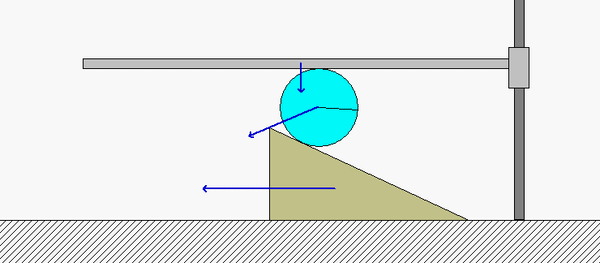# Homework H.2.GDiscussionPoints B and D on the disk roll without slipping on the horizontal guide A and the wedge E, respectively. Because of this:

• B has a velocity vB which points straight down, with the same speed vA as the horizontal guide A.
• D has a velocity vD which points directly to the left with the same speed as the wedge E.

The instant center (IC) of the disk (call it point C) therefore exists at the intersection of the perpendiculars to vand vD. Note that point B is much closer to the IC than point D. What does this say about the relative sizes of vD and vB? Is this consistent with the speeds of D and B shown in the above animation?

HINTS:

• Use the above discussion points to locate the IC for the disk.
• Determine the angular speed of the disk based on the speed of B and the distance from B to C.
• The speed of the wedge E is found by using the angular speed from above and the distance from C to D.

## 9 thoughts on “Homework H.2.G”

1.Paul V Richards says:

Why is the velocity at point D directly to the left, and not at an angle?

1.Flynn Botkin says:

This is because the disk is rolling without slipping, which means that any point making contact with another object must have the same velocity as that object. Therefore, as point D is making contact with wedge E, it must have the same velocity as wedge E, which is constrained horizontally.

2.Chance Anthony Lehman says:

I’m confused as to how to find the IC. Are we supposed to use Vd because Vd =Ve? And if that is the case, Using Vd causes the IC to be slightly off from point B so I don’t see what the next move is.

1.CMK says:

Chance: Once you locate the IC of the disk (let's call that point C), you can find the angular speed of the disk, ω_disk, by dividing the speed of B by the distance from C to B. And, in a similar way, the speed of D is then equal to ω_disk times the distance from C to D.

Does this help?

1.Chance Anthony Lehman says:

A little, I'm still confused as to where the IC should be though. Because if the IC is where I have it now, then I'm not really sure how to calculate the distance to point B or D.

3.jone1871 says:

Should we put the location of point C relative to the center of the disk?

1.CMK says:

jone1871: Yes, please give the location of the IC referenced to point O.

1.Matthew Torrisi says:

How should we reference it exactly? Right now I have 'a distance of ([x]i_hat + [y]j_hat) from point C.' Is that acceptable?

1.CMK says:

Yes, that would work. Or, simply show the location in a figure with the dimensions provided.